# Texas Go Math Kindergarten Lesson 4.6 Answer Key Understand Numbers Through 8

Refer to our Texas Go Math Kindergarten Answer Key Pdf to score good marks in the exams. Test yourself by practicing the problems from Texas Go Math Kindergarten Lesson 4.6 Answer Key Understand Numbers Through 8.

## Texas Go Math Kindergarten Lesson 4.6 Answer Key Understand Numbers Through 8

Unlock the Problem

DIRECTIONS: There are four buttons on the yellow raincoat. Trace the buttons. Draw more buttons for a total of six. Tell o friend about your drawing.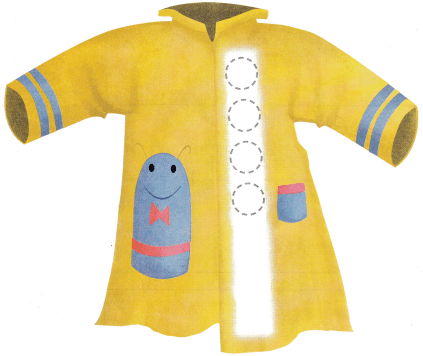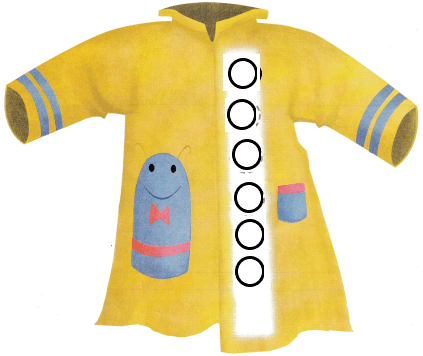Explanation:
There are four buttons on the yellow raincoat. I traced the buttons and drew 2 more buttons for a total of six.

Try Another Problem

DIRECTIONS: 1. George has 5 marbles. Alex has a number of marbles that is two greater than 5. Draw the marbles. Write the numbers. 2. Allison has 8 crayons. Jackie has the same number of crayons as Allison. Draw the crayons. Write the numbers.

Question 1.Explanation:
George has 5 marbles. Alex has a number of marbles that is two greater than 5 that is 7.
I drew 5 and 7 marbles in each box and wrote numbers 5 and 7.

Question 2.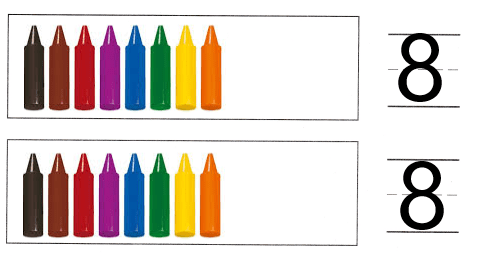Explanation:
Allison has 8 crayons. Jackie has the same number of crayons as Allison that is 8.
I drew 8 crayons in each box and wrote number 8.

Share and Show

DIRECTIONS: 3. Ben has 7 rocks. Molly has a number of rocks that is two less than 7. Draw the rocks. Write the numbers. 4. Jasmine has 4 stickers. Sadie has a number of stickers that is two more than 4. Draw the stickers. Write the numbers.

Question 3.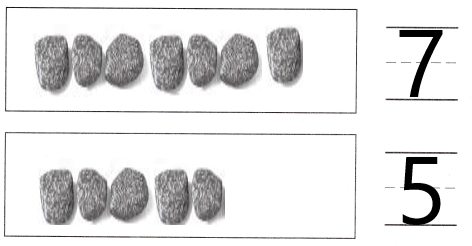Explanation:
Ben has 7 rocks. Molly has a number of rocks that is two less than 7 that is 5.
I drew 7 and 5 stones in each box and wrote numbers 7 and 5.

Question 4.Explanation:
Jasmine has 4 stickers. Sadie has a number of stickers that is two more than 4 that is 6.
I drew 4 and 6 stickers in each box and wrote numbers 4 and 6.

HOME ACTIVITY • Give your child 8 small items such as buttons. Have your child count the items and tell you how many.

DIRECTIONS: 5. Draw a set of blue kites that has two more than the red kites. 6. Draw a set of red kites that has the same number as the blue kites. 7. Choose the correct answer. Which group has one more than 7?

Question 5.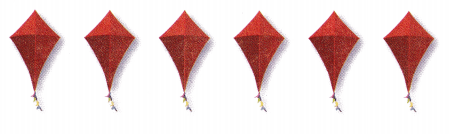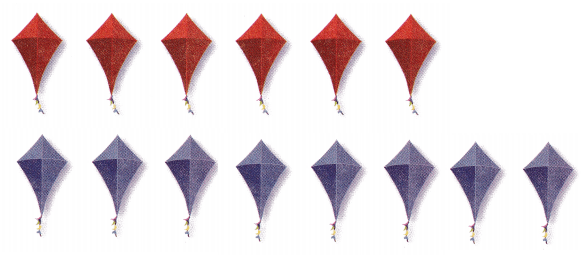Explanation:
There are 6 red kites.I drew 8 blue kites that is 2 more than the number of red kites.

Question 6.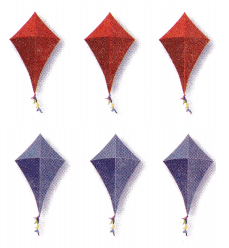Explanation:
There are 3 blue kites.So, i drew as many red kites as blue kites.I drew 3 red kites.

Question 7.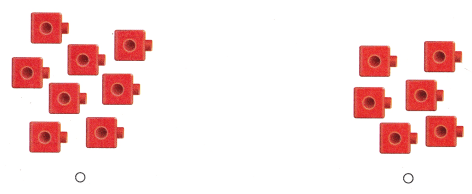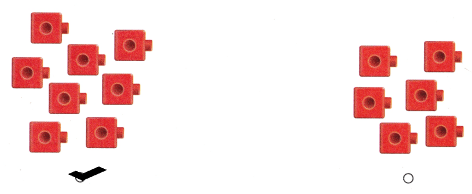Explanation:
I marked the group that has more number of cubes than 7.

### Texas Go Math Kindergarten Lesson 4.6 Homework and Practice Answer Key

DIRECTIONS: 1. Jeremy has 6 balloons. Chad has a number of balloons that is one more than 6. Draw the balloons. Write the numbers. 2. Diane has 8 pencils. Jill has a number of pencils that is two less than 8. Draw the pencils. Write the numbers.

Question 1.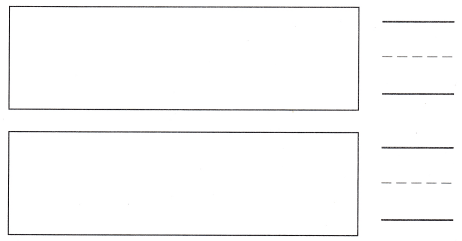Explanation:
Jeremy has 6 balloons. Chad has a number of balloons that is one more than 6 that is 7.
I drew 6 and 7 balloons in each box and wrote numbers 6 and 7.

Question 2.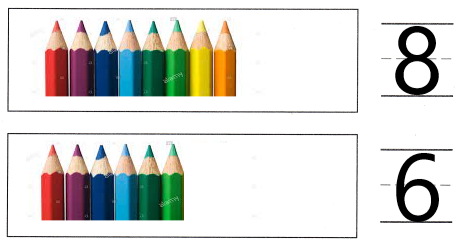Explanation:
Diane has 8 pencils. Jill has a number of pencils that is two less than 8 that is 6.
I drew 8 and pencils in each box and wrote numbers 8 and 6.

DIRECTIONS: Choose the correct answer. 3. There is a number of banners that is two more than 6. How many banners are there? 4. Joe has a number of hats that is two less than 8. How many hats does he have? 5. Which group has two more than 5?

Lesson Check

Question 3.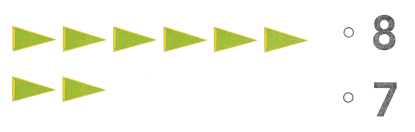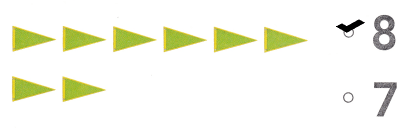Explanation:
There is a number of banners that is two more than 6 that is 8.So, i marked the number 8.

Question 4.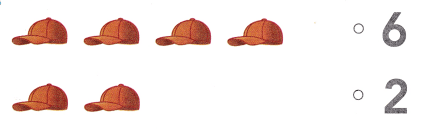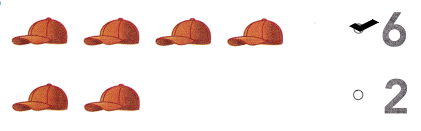Explanation:
Joe has a number of hats that is two less than 8 that is 6.So, i marked 6.

Question 5.